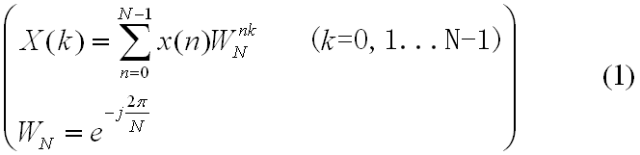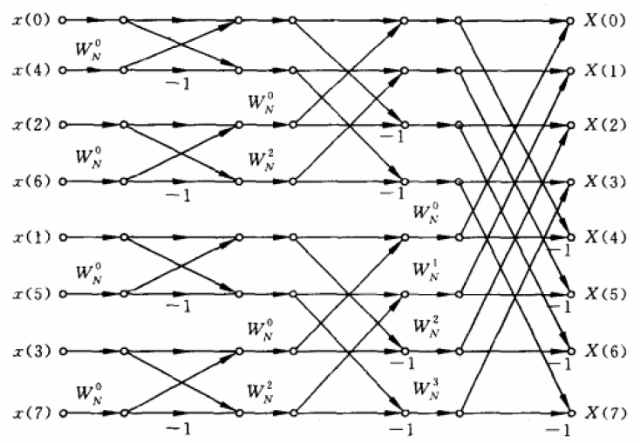•FFT
• 数字信号快速傅里叶算法实验设计。用MATLAB实现画序列及离散频谱图。
• 通过编程实现蝶形快速傅里叶算法void fft ; 快速傅里叶变换 void initW ; 初始化旋转因子 void change ; 变址 复数运算 void add complex complex complex ; void mul complex complex complex ; void sub ...
• split_step_fourier_method_split_split-stepfourier_opticalfiber_分布傅里叶_分布傅里叶算法_源码.rar
• matlab语言 分布傅里叶算法 +原始论文Optimization of the Split-Step Fourier Method in Modeling Optical-Fiber Communications Systems
• 快速傅里叶算法fftw源代码，采用各种优化技术如SIMD等
• 周期信号 全波 傅里叶算法 微机继电保护 电力系统
• 用STM32系列芯片做傅里叶变换的算法和程序，希望对大家有帮助
• FFT是一种DFT的高效算法，称为快速傅立叶变换（fast Fouriertransform）。FFT基本上可分为两类，时间抽取法和频率抽取法，而一般的时间抽取法和频率抽取法只能处理长度N=2^M的情况，另外还有组合数基四FFT来处理一般...
• 提出了一种应用快速傅里叶变换算法提高哈特曼夏克波前传感器波前重构实时性的快速算法。在根据波前斜率值应用最小二乘法估计波前相位的过程中,应用快速傅里叶变换算法进行方程的对角化和相位值的解耦,算法精度高、...
• 离散形式的傅里叶变换可以利用数字计算机快速地算出
• 利用MATLAB工具对改进傅里叶算法和传统傅里叶算法的计算结果进行仿真，由仿真结果可知，在不考虑非整次谐波分量影响时，改进傅里叶算法对滤除电流、电压故障信号中的衰减直流分量的效果十分理想。在此基础上，通过...
• 用c语言实现的快速傅里叶算法，用了三个大循环，时域乱序输入频域自然序输出FFT
• 关于图像匹配的很好的算法，可以用来实现，是非常好的一个算法
•FFT
• 快速傅里叶算法（C语言）  考研阶段学习过傅里叶级数，而最近的项目正好是用C语言编写傅里叶变换，于是很认真的复习了傅里叶级数。可是无奈，看来看去反而晕晕乎乎的。后经师兄师姐的指教，才得知对于工程中的信号...

快速傅里叶算法（C语言）

考研阶段学习过傅里叶级数，而最近的项目正好是用C语言编写傅里叶变换，于是很认真的复习了傅里叶级数。可是无奈，看来看去反而晕晕乎乎的。后经师兄师姐的指教，才得知对于工程中的信号处理，研究周期性的傅里叶变换都没有现实意义，而傅里叶级数更没有什么关系。        工程中待处理的信号，通常具有非周期性，故我们需要对离散傅里叶变换进行研究。离散公式：【x(n)是采样的时域信号，X(k)是对于不同频率k的频域信号。】

而快速傅里叶变换又是对离散傅里叶变换的改进，通过蝶形运算（网上的图片如下），计算速度可大大提升，使计算量呈指数型下降。【最左的x(n)是采样的时域信号，最右的X(k)是算出的频域信号。可以看到左边的x(n)中，n 的序列并非正常的递增，这是为了使得输出的X(k)中的k频率呈递增序列。[n]的序列是通过将十进制n转化成的二进制数字后，倒序排列生成的，如4的二进制码为100，倒序为001，故排在第二位。在上图中，共8个信号，可分成3级。第一级，每个分组两个节点，一个蝶形单元。第二级，每个分组四个节点，两个蝶形单元。第三级，每个分组八个节点，四个蝶形单元。】   核心代码：
<span style="font-size:12px;">void fft(complex f[], int N)
{
complex t, wn;//中间变量
int i, j, k, la, lb, lc, l, r, m, n;//中间变量
//M:分解运算级数
int M;
/*----计算分解的级数M=nextpow2(N)----*/
for (i = N, M = 1; (i = i / 2) != 1; M++);
/*----输入信号二进制码位倒序*/
for (i = 1, j = N / 2; i <= N - 2; i++)
{
if (i<j)
{
t = f[j];
f[j] = f[i];
f[i] = t;
}
k = N / 2;
while (k <= j)
{
j = j - k;
k = k / 2;
}
j = j + k;
}

/*----FFT算法----*/
for (m = 1; m <= M; m++)
{
la = pow(2.0, m); //la=2^m代表第m级每个分组所含节点数
lb = la / 2;    //lb代表第m级每个分组所含碟形单元数
//同时它也表示每个碟形单元上下节点之间的距离
/*----碟形运算----*/
for (l = 1; l <= lb; l++)
{
r = (l - 1)*pow(double(2), M - m);
for (n = l - 1; n<N - 1; n = n + la) //遍历每个分组，分组总数为N/la
{
lc = n + lb;  //n,lc分别代表一个碟形单元的上、下节点编号
Wn_i(N, r, &wn, 1);//wn=Wnr
c_mul(f[lc], wn, &t);//t = f[lc] * wn复数运算
c_sub(f[n], t, &(f[lc]));//f[lc] = f[n] - f[lc] * Wnr
c_plus(f[n], t, &(f[n]));//f[n] = f[n] + f[lc] * Wnr
}
}
}
}</span>


展开全文• 目前最快的快速傅里叶算法，能够有效提高傅里叶变换速度FFTW
• 文件是一个使用C语言实现的快速傅立叶算法，C语言编程，在DSP环境下实现的，适合初学者的，可用与DSP等信号处理领域。
• 为提高模拟超连续谱(SC)产生的精度, 在不增加计算量的情况下对传统分步傅里叶算法作了一定的改进。传统分步傅里叶算法只考虑初始脉冲中心频率对应的色散系数, 而超连续谱的光谱范围很大, 当色散系数随波长变化较大时...
• ARM7 lpc2132 利用快速傅里叶算法实现电容测量功能源代码
• FFT算法详解，深入浅出。易学易懂。 鉴于现在FFT算法资料的复杂难懂，本文由浅入深循序渐进的讲解了FFT算法的细节，让FFT不在晦涩难懂。FFT
• 描述光脉冲在光纤中传输的物理过程是“非线性薛定谔方程”，该代码是基于matlab对非线性薛定谔方程的数值求解，用到的算法为分步傅里叶算法
• So the problem we need to solve is that of multiplying polynomials fast. Doing it the regular convolution way takes quadratic time -O(n2). This is OK for small n but won't do for large n. ...
• 考研阶段学习过傅里叶级数，而最近的项目正好是用C语言编写傅里叶变换，于是很认真的复习了傅里叶级数。可是无奈，看来看去反而晕晕乎乎的。后经师兄师姐的指教，才得知对于工程中的信号处理，研究周期性的傅里叶...
      一、基础知识
考研阶段学习过傅里叶级数，而最近的项目正好是用C语言编写傅里叶变换，于是很认真的复习了傅里叶级数。可是无奈，看来看去反而晕晕乎乎的。后经师兄师姐的指教，才得知对于工程中的信号处理，研究周期性的傅里叶变换都没有现实意义，而傅里叶级数更没有什么关系。        工程中待处理的信号，通常具有非周期性，故我们需要对离散傅里叶变换进行研究。离散公式：【x(n)是采样的时域信号，X(k)是对于不同频率k的频域信号。】
而快速傅里叶变换又是对离散傅里叶变换的改进，通过蝶形运算（网上的图片如下），计算速度可大大提升，使计算量呈指数型下降。一、最左的x(n)是采样的时域信号，最右的X(k)是算出的频域信号。可以看到左边的x(n)中，n 的序列并非正常的递增，这是为了使得输出的X(k)中的k频率呈递增序列。
二、[n]的序列是通过将十进制n转化成的二进制数字后，倒序排列生成的，如4的二进制码为100，倒序为001，故排在第二位。
三、在上图中，共8个信号，可分成3级。第一级，每个分组两个节点，一个蝶形单元。第二级，每个分组四个节点，两个蝶形单元。第三级，每个分组八个节点，四个蝶形单元。
二、算法语言实现
下面是一段HTML快速傅里叶核心代码，和C语言差不多：
<span style="font-size:12px;">

void fft(complex f[], int N)
{
complex t, wn;//中间变量
int i, j, k, la, lb, lc, l, r, m, n;//中间变量
//M:分解运算级数
int M;
/*----计算分解的级数M=nextpow2(N)----*/
for (i = N, M = 1; (i = i / 2) != 1; M++);
/*----输入信号二进制码位倒序*/
for (i = 1, j = N / 2; i <= N - 2; i++)
{
if (i<j)
{
t = f[j];
f[j] = f[i];
f[i] = t;
}
k = N / 2;
while (k <= j)
{
j = j - k;
k = k / 2;
}
j = j + k;
}
/*----FFT算法----*/
for (m = 1; m <= M; m++)
{
la = pow(2.0, m); //la=2^m代表第m级每个分组所含节点数
lb = la / 2;    //lb代表第m级每个分组所含碟形单元数
//同时它也表示每个碟形单元上下节点之间的距离
/*----碟形运算----*/
for (l = 1; l <= lb; l++)
{
r = (l - 1)*pow(double(2), M - m);
for (n = l - 1; n<N - 1; n = n + la) //遍历每个分组，分组总数为N/la
{
lc = n + lb;  //n,lc分别代表一个碟形单元的上、下节点编号
Wn_i(N, r, &wn, 1);//wn=Wnr
c_mul(f[lc], wn, &t);//t = f[lc] * wn复数运算
c_sub(f[n], t, &(f[lc]));//f[lc] = f[n] - f[lc] * Wnr
c_plus(f[n], t, &(f[n]));//f[n] = f[n] + f[lc] * Wnr
}
}
}
}
</span>


展开全文...